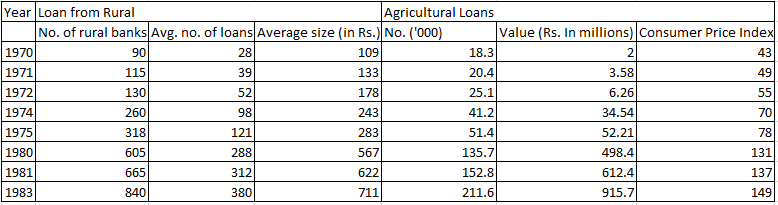### CAT 1997 Question Paper Question 23

Instructions

The table given below gives the annual details of loans from rural banks and agricultural loans over the years 1970 to 1983. Using this data answer the questions that follow.Question 23

# In 1974, the amount of agricultural loans formed what percentage of the total loans?

Solution

Total loan from rural in 1974 = $$260 \times 98 \times 243$$ = 6 million (nearly)

Loan for agriculture in 1974 = 34.5 million

Total = 34.5 + 6 = 40.5

%Agriculture loan = $$\frac{34.5}{40.5} \times 100$$ = 85% (Nearly)

• All Quant CAT Formulas and shortcuts PDF
• 30+ CAT previous papers with solutions PDF